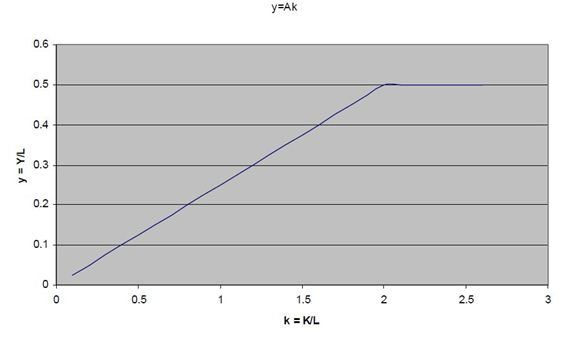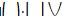The Harrod Domar Model

Main Prediction: GDP growth is proportional to the share of investment spending in GDP.

Assumptions:

1. Assume unemployed labor, so there is no constraint on the supply of labor.

2. Production is proportional to the stock of machinery.

Growth Rate of GDP

We want to determine the growth rate of GDP, which is defined as:

G(Y) = (change in Y) / Y where Y = GDP

To do this, we estimate the Incremental Capital-Output Ratio (ICOR), which is a measure of capital efficiency.

ICOR = (change in K) / (change in Y) where K = capital stock

A high ICOR implies a high increase in capital stock relative to the increase in GDP. Thus, the higher the ICOR, the lower the productivity of capital.

Since capital is assumed to be the only binding production constraint, investment (I) in the Harrod-Domar model is defined as the growth in capital stock.

I = (change in K)

But investment is also equal to savings (S), which is equal to the average propensity to save (APS) times GDP (Y). Denote APS = s

I = S = APS * Y = s*Y

So,

ICOR = (s Y) / (change in Y)

Rearranging terms,

G(Y) = (change in Y) / Y = s / ICOR

Growth Rate of GDP per Capita

The growth rate of GDP per Capita is defined as

G(Y/P) = G(Y) – G(P) where G(P) = the population growth rate

From (1),

G(Y/P) = s / ICOR – G(P)Thus, a 1 percent increase in population growth will cause the growth rate of GDP per capita to decrease by 1 percent.  The empirical question is whether policy makers can achieve a constant marginal product of capital when the centralize investment decisions.

Examples

1. Assume that a country has a savings/investment rate of 4 percent of their GDP and an ICOR of 4, they will have a growth rate of 1 percent.  But if the population growth rate were also 1 percent, then the country would have zero GDP growth per capita.  These assumptions imply that for a country to develop, it needed to have an investment rate of around 12-15 percent of GDP, which would result in a GDP growth rate of 3 percent. The country in our example, however, only invests 4 percent.  The difference between the required investment rate (12 percent) and the country’s own investment (4 percent) is what development economists call the financing gap. Foreign aid by Western countries fills this financing gap in order to attain the target growth for the country. However, unless the investment is used productively, there is no guarantee that it will generate the desired change in output.

2. The Harrod-Domar model assumes fixed coefficients:

Y = F(K,L) = min (AK,BL) where

A and B are positive constants. With fixed proportions, if the available capital and labor happen to fit AK=BL, then all workers and equipment are fully employed. However, if AK>BL, then only BLAof the capital is used and the rest is unemployed. If AK<BL, then only AKBof the labor is used and the rest is unemployed.

3. Assume that Shanistan producers cigars with a fixed coefficient technology:

Y = min (1/4K, 1/2L)

Currently, Shanistan has 80 units of K and 100 L. What is the feasible output?

Y = 20

What is employment? (1/2) 80 = 40

Divide both sides by L to get per capita output:

y = min (Ak, B)

If k< B/A, then K is fully employed and output y = Ak

For k> B/A, then Y = BL and capital would be unemployed, so y = B.

(In the graph, below, we derive y and k when 0<K<30 and L = 10).

Limitations of the model:

1.   Economic growth and economic development are not the same. Economic growth is a necessary but not sufficient condition for development

2.   Harrod Domar model was formulated primarily to protect the developed countries from chronic unemployment, and was not meant for developing countries.

3.   Practically it is difficult to stimulate the level of domestic savings particularly in the case of LDCs where incomes are low.

4.   It fails to address the nature of unemployment exists in different countries.  In developed countries, the unemployment is ‘cyclical unemployment’, which is due to insufficient effective demand; whereas in developing countries, there is ‘disguised unemployment’.

5.   Borrowing from overseas to fill the gap caused by insufficient savings causes debt repayment problems later.

6.   The law of diminishing returns would suggest that as investment increases the productivity of the capital will diminish and the capital to output ratio rise.

The Harrod-Domar model of economic growth cannot be rejected on the ground of above limitations.  With slight modifications and reinterpretations, it can be made to furnish suitable guidelines even for the developing economies.